RotationNet in fast.ai: Adventures in Virtual Set Creation and Computer Vision

Over the long story that is my career as an environment artist working for video game and virtual reality developers, I’ve spent many tedious hours gathering and creating resources to populate virtual sets. I can imagine a time in our near future where all that we need to create a fully-realized virtual world is to provide to an A.I. assistant a description of what we want, a few drawings, photographs, or videos showing some ideas, and in a short time this digital assistant would give the user an environment reflecting those ideas. Think something like the Holodeck from Star Trek. My recent explorations into neural networks and machine learning suggest to me that we are quickly approaching this reality.

One of the first pieces of technology we would need to achieve this sci-fi fantasy is object classification and pose estimation. There are several approaches to this problem, but the one I decided to study and implement is RotationNet: Joint Object Categorization and Pose Estimation Using Multiviews from Unsupervised Viewpoints by Asako Kanezaki , Yasuyuki Matsushita and Yoshifumi Nishida. Their published paper can be found at https://arxiv.org/abs/1603.06208. I found their description in the paper difficult to follow, but they’ve published a github repository of a PyTorch implementation, found here: https://github.com/kanezaki/pytorch-rotationnet. I dug deeply into the code in an attempt to understand it’s methodologies and to explore if this would be a viable approach to achieving a small but important part of automated virtual set creation. These are my findings.

RotationNet

The basic idea behind RotationNet is to train a CNN using a fixed number of images that represent an object rotated in a specific, predetermined order. During training, the pose (i.e. the angle from which the image was captured) is discovered as a latent feature, meaning that it is determined through unsupervised learning. After training, anywhere from 1 to the total number of images in a single training datapoint can be fed into the CNN, and the object’s category and pose will be predicted.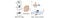From the RotationNet paper, showing inference. Feed a series of images representing different views of the object into the trained RotationNet CNN. It will predict both an object category and the view the image came from (the pose).

Training involves a CNN pre-trained on Imagenet, but the output is customized and then processed to determine the view order, which is the latent feature discovered during training.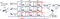From the RotationNet paper, showing the training process. A category label (car) and a full set of images (3 in this example, in a predetermined rotation order) are fed into the CNN. For each image the CNN outputs as many groups of activations as there are images (3, again). Each group contains one activation for each object category (2 in this example: car and bed), plus an extra activation for a “background” category, meaning it corresponds to none of the object categories. Softmax is performed on each group of activations. Depending on the view setup, there will be a specific number of view rotation candidates. For this example there are three: (1,2,3), (2,3,1) and (3,1,2). A “score” is calculated for each candidate and the candidate with the highest score is selected as the “best” view, meaning it’s the group of views that have the largest softmax values for the category label ((2,3,1) in this example). A target label is then created which consists of the category label in the position of its highest softmax value and the “background” category in the remaining positions. Cross-entropy loss is calculate using this new target label and the original output of the CNN before backpropagation is performed.

Processing the CNN Output to Generate the Target Tensor

As mentioned earlier, I found it difficult to completely grasp the training process through reading the paper. Thanks to fast.ai I understand how to setup and train a CNN, but the post-CNN processing was difficult for me to understand. The PyTorch implementation code is, thankfully, very concise and clear, and stepping through it really helped me to understand what they are doing. It was also helpful for me to create illustrations of the various tensors as they were created, reshaped, and updated. I present here my illustrations and commentary.

An Imagenet pretrained CNN (AlexNet in my implementation) is adjusted so that it will output a large two-dimensional tensor. The first dimension of this tensor needs to be the number of samples that are being simultaneously input into the CNN (the minibatch size) times the number of training images per datapoint. The second dimension needs to be the number of classes plus one (the extra “background” class) times the number of training images per datapoint. It is then reshaped so that the classes align along the first dimension, as illustrated below.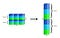Left: Illustration representing the two-dimensional tensor output by the CNN. In this example there are two samples in the minibatch, two classes (the two darker shades of each color), and three training images per sample (blue, cyan, and green). Notice that an extra class is added (the “background” class represented by the lightest shade of each color). Right: The tensor is then reshaped so that each class is aligned along the second dimension.

This reshaped tensor is what will later be used during the cross-entropy loss calculation.

A softmax calculation is then performed along the columns to scale and normalize the activations. The next step consists of subtracting the “background” class from each of the other classes, and then discarding it. I don’t think this step is described in the paper, but what I think this is doing is weakening the softmax values that have a strong “background” class activation, thus making the softmax values for the desired class label relatively stronger. The resulting tensor is then reshaped a couple more times so that the final tensor’s first dimension is class, second is image, and third is sample. These reshapings are setting up the tensor for the “score” calculation that will determine which combination of views has the strongest softmax values.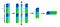Setting up the tensor for the “score” calculation. After performing softmax along the second dimension, the last column (the “background” class) is subtracted from the other columns and discarded, resulting in an 18x2 tensor. That is then reshaped so that the samples stack in the third dimension, then reshaped again so the class is the first dimension, image is the second, and sample is the third.

Next, the “score” for each view candidate is calculated. For each sample, the tensor values from the previous step are added together for each view candidate and stored in the score tensor. The resulting score tensor contains values that represent which view candidates have the strongest softmax values. Another one-dimensional tensor is created that will contain the target classes. It’s initialized with the “background” class. From the score tensor the view candidate that has the highest score is selected, and the target class tensor is populated with the target label in the positions determined by this selection.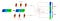Creating the target class tensor. A score tensor (red, illustrated with its 2nd and 3rd dimensions exploded, for clarity) is created. Its dimensions are the number of classes by the number of view candidates by the number of samples (2x3x2 in this example). For each view candidate, the softmax values are added together and stored in the score tensor. For each sample, the element of the score tensor that has the highest value is selected (In this example 0 for the first sample and 2 for the second). Finally, the target class tensor, which has been initialized to the “background” class (2 in this example), is populated with the target class in the positions determined by the selected view candidate. The target classes in this example are 0 for first sample and 1 for the second.

Now that we have our target tensor, loss can be calculated and backpropagation performed.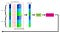Calculating loss. The target class tensor and the initial reshaping of the CNN output are used to calculate loss for each image and view. Average loss is calculated for training evaluation, then standard backpropagation is performed.

fast.ai Implementation

I worked through the first part of the fast.ai MOOC classes taught by Jeremy Howard, and attended the in-person classes earlier this spring. I’m really excited about his approach and the libraries that simplify and streamline the whole process of training a neural network. To help dig deep into their libraries and develop my understanding of them I tried to work within the fast.ai ecosystem as much as possible. I was mostly successful, but there were a few complications and I did need to alter their library code slightly to account for the differences the RotationNet implementation requires. I’ll go into those in more detail below.

For those not familiar with fast.ai, it’s an excellent collection of libraries built on top of PyTorch that streamline and encode a lot of the current best practices for deep learning. It currently supports various deep learning techniques, including CNN’s, RNN’s, U-nets, tabular data, NLP, etc. I’ve been having a lot of fun playing around with it over that past few months to develop some computer vision ideas (i.e. https://github.com/amathis726/self-steering-ue4-beta), and this project is my attempt to dig deeper into their libraries and achieve a greater understanding, as well as develop some cool computer vision tools that might have some useful applications down the road.

To keep things as flexible as possible, fast.ai has implemented into its training loop the concept of “callbacks,” which are basically just a way to insert some custom functionality into the training loop at various predetermined points in the training process. Because RotationNet does extensive processing to the output tensor of the CNN before calculating the loss I knew I’d have to implement a few callbacks. Specifically, I created four with significant functionality:

1) On_epoch_begin

Because each datapoint of the training dataset consists of multiple images, I couldn’t shuffle each minibatch randomly, otherwise the image groupings would be lost and the latent view variable would not be able to be determined. Yet, when I tried training the CNN with an unshuffled minibatch I was unable to achieve accuracies above 30%. Thus, at the beginning of each epoch, I had to implement a custom shuffle routine which shuffled the order of groups of images in a minibatch, but kept the groupings and their internal order intact.

2) On_loss_begin

This callback is called immediately after a minibatch has worked its way through the CNN, but before the loss is calculated and backpropagation begins. This is the exact time that I needed to process the output tensor (process illustrated above) to generate the target class tensor.

3) On_batch_end

The fast.ai libraries have a system built in to automatically calculate loss and accuracy, and display it to the user in a nice table. It works beautifully for most deep learning applications, but RotationNet needs accuracy not just for a single image, but for a group of images. This callback is called right before the validation phase for a minibatch, so this is the perfect point to call my own customized validation scoring.

4) On_epoch_end

This callback is called at the end of each epoch. Because RotationNet needs customized metrics, this is the point when its custom losses and accuracy are calculated and displayed to the user.

fast.ai Source Code Changes

Because RotationNet has a few requirements that set it apart from other image classification tasks, I had to make some very minor changes to fast.ai’s library source code. Specifically, in the training loop, before calculating loss, I needed to process the CNN output and generate the target class tensor. Fast.ai’s on_loss_begin callback only returns one argument, so I had to add another to make sure the target class tensor got saved for future use. Also, during the routine to calculate loss I also needed to add a check to see if the model was evaluating or training. If evaluating it shouldn’t create the target class tensor because the view variable is latent and discovered during training, and thus shouldn’t be evaluated at validation time.

Results

The authors of the RotationNet paper created their own dataset, called MIRO, to prove that their approach has real-world applications. It consists of 12 classes, with 9 training datapoints for each class, and one validation datapoint for each class. Obviously this a very tiny dataset, but I think it was successful in showing that RotationNet’s approach is feasible and it’s able to make accurate predictions for both classification and pose. Here are a few examples of my results using MIRO: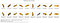Predictions for class and pose for a random set of images from one datapoint in the validation set. Top: input images. Bottom: representative image from the training set showing predicted class and pose.

The top row shows a random set of images from one datapoint in the validation set. The bottom row shows a representative image from the training set, showing predicted class and pose. The final printout at the bottom shows the ultimate class prediction, which is simply the class that had the most predictions. For the example above, there were 15 total images input into the CNN for evaluation (only the first 10 are shown). Since “bus” was the overwhelming majority of predictions, it’s final prediction is “bus”.

Notice that the predicted pose is actually a number, not an image, as might be incorrectly inferred from my graphic. The images in the second row are only a graphical representation of the pose number. Because “pose 13” is meaningless and/or difficult to decipher for us humans, it’s easier to validate results when represented this way.

The pose number represents the view that was determined during training. The view that had the strongest “score” during training is designated as the first pose (pose 0), and, because the views are captured in a predetermined rotation order, the pose numbers that follow the first pose follow this same order. As a simple example, let’s assume there were only three views in the training data. Since the views are created in a predetermined rotation order (either clockwise or counter-clockwise) then there can be only three view candidates ((1,2,3), (2,3,1), and (3,1,2)). When the first pose is determined during the scoring phase the pose order that follows is determined at the same time (i.e. if the first pose is 1 then the order is 1,2,3, if the first pose is 2 then the order is 2,3,1, etc.).

More MIRO examples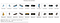Even though it misclassified several of these images, the ultimate prediction was correct because there were many input images that it did classify correctly. This illustrates one of the strengths of RotationNet in that the more images provided of the object the more accurate its classification will be. Note where the pose estimations are close but wrong (1, 2, 5, 8, 10). It might be interesting to explore validation sets where the input images are closer together in pose and see if that improves pose estimates. Case 7 is particularly interesting in that the pose is nearly 180 degrees off, suggesting that the CNN has difficulty distinguishing front from back for this particular view of this object.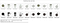Extremely accurate pose estimates. I suspect that having a strong landmark like the handle helps accomplish this.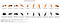Fairly good pose prediction. Similarly to the car example above, it confuses front and back in 5 and 6.

Difficult Case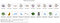The CNN has a very difficult time with these images. It gets most of both the classifications and poses wrong. It is, however, able to predict the ultimate classification correctly. Further exploration is needed as to why the CNN has such a difficult time with this object. Maybe a better pre-trained CNN would be helpful. Current implementation uses AlexNet.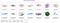Even though only provided a small number of input images and predicting the class mostly wrong, the CNN was able to ultimately correctly predict the class.

Final Words

The results are encouraging. This seems like a valid approach to object recognition and pose estimation. After implementing object detection functionality I can imagine providing a piece of concept art, or a video or photos of a set walk-through and having the CNN recognize the objects represented in it. Theoretically, it should then be possible to pull up models from a database and be able to orient them similarly to how they are positioned in the representation.

Thanks for reading. Ideas, feedback, and suggestions are always welcome.

Code can be found at: https://github.com/amathis726/rotationNet

Written by